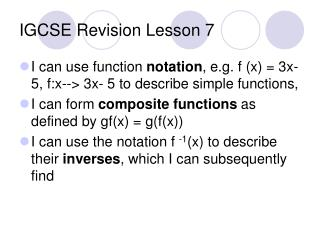DownloadDownload PresentationIGCSE Revision Lesson 7

# IGCSE Revision Lesson 7

Download Presentation## IGCSE Revision Lesson 7

- - - - - - - - - - - - - - - - - - - - - - - - - - - E N D - - - - - - - - - - - - - - - - - - - - - - - - - - -
##### Presentation Transcript

1. IGCSE Revision Lesson 7 • I can use function notation, e.g. f (x) = 3x- 5, f:x--> 3x- 5 to describe simple functions, • I can form composite functions as defined by gf(x) = g(f(x)) • I can use the notation f -1(x) to describe their inverses, which I can subsequently find

2. IGCSE Revision Lesson 7 f(x) means “some function applied to x” e.g. f(x) = 3x + 2 f(2) = f(4) = f(-1)=

3. IGCSE Revision Lesson 7 Notice the difference: f(x) = 3x + 2 f(2) = f(x) = 5, x = We are working backwards. “When you do ‘f’ to x, the answer is 5. What is x?’

4. IGCSE Revision Lesson 7 • We also have f(x) = 3x + 2 f -1(4) = f -1 (x) =

5. IGCSE Revision Lesson 7 e.g. g(x) = 4(x – 5) g(1) = g(x) = 16 g -1(20) = g -1(x) = 2 What equivalent statements can we make for these?

6. This notation can also be used in graphs...

7. IGCSE Revision Lesson 7 f(x) = x2 + 2 g(x) = 3x – 1 fg(x) = gf(x) = Read as f(g(x)) Read as g(f(x)) fg(x) = f(3x – 1) gf(x) = g(x2 + 2) = (3x – 1)2 + 2 = 3(x2 + 2) -1 = 9x2 – 6x + 1 + 2 = 3x2 + 6 – 1 = 9x2 – 6x + 3 = 3x2 + 5

8. IGCSE Revision Lesson 7 fg(x) = gf(x) = gh(x) = ff(x) =

9. Inverse – working the other way. e.g. f(x) = 3x – 2 Method 1: Flow diagram What does f(x) tell us to do to x? Method 2: Change the terms x = input, let y = output Inverse means we switch the input and output... then solve. y = 3x – 2  x = 3y – 2 x + 2 = 3y x + 2 = y 3 IGCSE Revision Lesson 7 • Method 2: Change the terms x = input, let y = output Inverse means we switch the input and output... then solve. y = 3x – 2  x = 3y – 2 x + 2 = 3y x + 2 = y 3 x 3 - 2 x 3x 3x - 2 ÷ 3 + 2 x + 2 x + 2 x 3